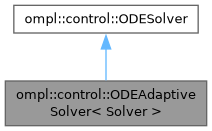ompl::control::ODEAdaptiveSolver< Solver > Class Template Reference

Adaptive step size solver for ordinary differential equations of the type q' = f(q, u), where q is the current state of the system and u is a control applied to the system. The maximum integration error is bounded in this approach. Solver is the numerical integration method used to solve the equations, and must implement the error stepper concept from boost::numeric::odeint. The default is a fifth order Runge-Kutta Cash-Karp method with a fourth order error bound. More...

#include <ompl/control/ODESolver.h>

Inheritance diagram for ompl::control::ODEAdaptiveSolver< Solver >:[legend]

## Public Member Functions

ODEAdaptiveSolver (const SpaceInformationPtr &si, const ODESolver::ODE &ode, double intStep=1e-2)
Parameterized constructor. Takes a reference to the SpaceInformation, an ODE to solve, and an optional integration step size - default is 0.01.

double getMaximumError () const
Retrieve the total error allowed during numerical integration.

void setMaximumError (double error)
Set the total error allowed during numerical integration.

double getMaximumEpsilonError () const
Retrieve the error tolerance during one step of numerical integration (local truncation error)

void setMaximumEpsilonError (double error)
Set the error tolerance during one step of numerical integration (local truncation error)Public Member Functions inherited from ompl::control::ODESolver
ODESolver (SpaceInformationPtr si, ODE ode, double intStep)
Parameterized constructor. Takes a reference to SpaceInformation, an ODE to solve, and the integration step size.

virtual ~ODESolver ()=default
Destructor.

void setODE (const ODE &ode)
Set the ODE to solve.

double getIntegrationStepSize () const
Return the size of a single numerical integration step.

void setIntegrationStepSize (double intStep)
Set the size of a single numerical integration step.

const SpaceInformationPtrgetSpaceInformation () const
Get the current instance of the space information.

## Protected Member Functions

void solve (StateType &state, const Control *control, double duration) const override
Solve the ordinary differential equation given the input state of the system, a control to apply to the system, and the duration to apply the control. The value of state will contain the final values for the system after integration.

## Protected Attributes

double maxError_
The maximum error allowed when performing numerical integration.

double maxEpsilonError_
The maximum error allowed during one step of numerical integration.Protected Attributes inherited from ompl::control::ODESolver
const SpaceInformationPtr si_
The SpaceInformation that this ODESolver operates in.

ODE ode_
Definition of the ODE to find solutions for.

double intStep_
The size of the numerical integration step. Should be small to minimize error.Public Types inherited from ompl::control::ODESolver
using StateType = std::vector< double >
Portable data type for the state values.

using ODE = std::function< void(const StateType &, const Control *, StateType &)>
Callback function that defines the ODE. Accepts the current state, input control, and output state.

using PostPropagationEvent = std::function< void(const base::State *, const Control *, double, base::State *)>
Callback function to perform an event at the end of numerical integration. This functionality is optional.Static Public Member Functions inherited from ompl::control::ODESolver
static StatePropagatorPtr getStatePropagator (ODESolverPtr solver, const PostPropagationEvent &postEvent=nullptr)
Retrieve a StatePropagator object that solves a system of ordinary differential equations defined by an ODESolver. An optional PostPropagationEvent can also be specified as a callback after numerical integration is finished for further operations on the resulting state.

## Detailed Description

### template<class Solver = odeint::runge_kutta_cash_karp54<ODESolver::StateType>> class ompl::control::ODEAdaptiveSolver< Solver >

Adaptive step size solver for ordinary differential equations of the type q' = f(q, u), where q is the current state of the system and u is a control applied to the system. The maximum integration error is bounded in this approach. Solver is the numerical integration method used to solve the equations, and must implement the error stepper concept from boost::numeric::odeint. The default is a fifth order Runge-Kutta Cash-Karp method with a fourth order error bound.

Definition at line 273 of file ODESolver.h.

The documentation for this class was generated from the following file: